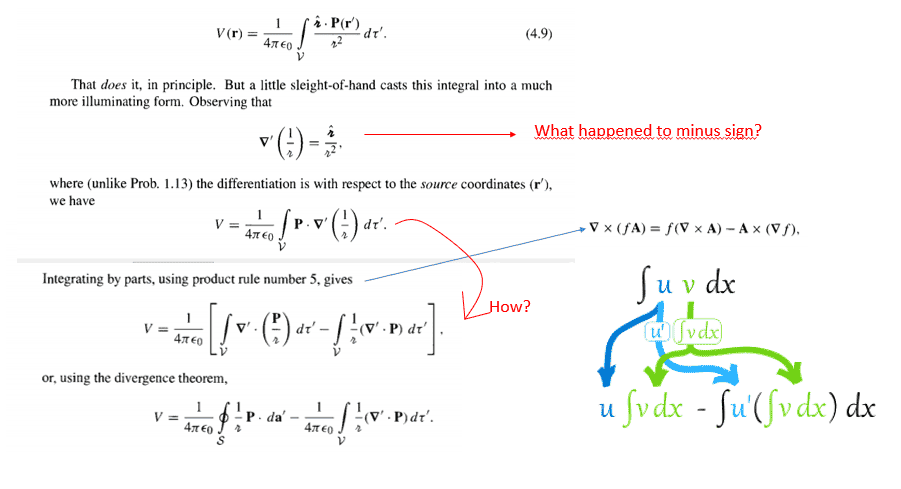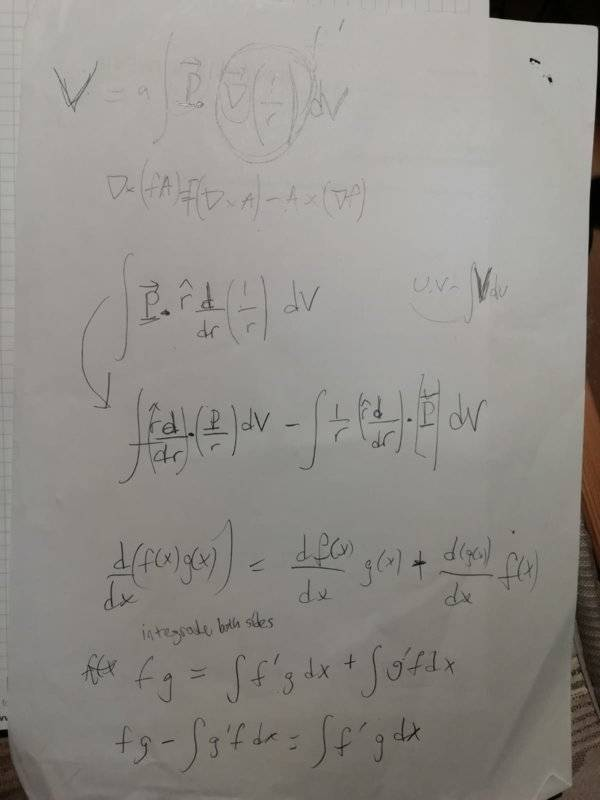# How to find an expression for bound charge densities in griffiths

• MrMuscle

#### MrMuscleAttempt:•Delta2
The minus sign is lost because the differentiation is with respect to the source coordinates ##r'##. It is a well known identity that $$\nabla\frac{1}{|\vec{r}-\vec{r'}|}=-\nabla'\frac{1}{|\vec{r}-\vec{r'}|}$$

where in the above the second ##\nabla## (the one in the right) has a ##'## next to it which means just that the differentiation is with respect to ##r'## coordinates.

For the rest you got the wrong identity involving curl and cross product. You should have the identity involving divergence and dot product which is as follows
$$\nabla\cdot (f\vec{A})=f\nabla\cdot\vec{A}+\vec{A}\cdot\nabla f$$

this identity holds for differentiating with source coordinates as well that is with ##\nabla'## in the place of ##\nabla##. Apply this Identity for ##\vec{A}=\vec{P}## and ##f=\frac{1}{|\vec{r}-\vec{r'}|}##.

Last edited:
•vanhees71 and MrMuscle

## 1. What is the concept of bound charge densities in Griffiths?

Bound charge densities refer to the electric charges that are bound to the atoms or molecules in a material and are not free to move. They arise due to the displacement of electrons from their equilibrium positions in the presence of an external electric field.

## 2. How do you calculate bound charge densities in Griffiths?

The expression for bound charge densities can be calculated by taking the divergence of the electric displacement field, which is related to the electric field by the permittivity of the material. This can be expressed as: ρb = -∇ · D = -∇ · (ε0εrE), where ρb is the bound charge density, ε0 is the vacuum permittivity, εr is the relative permittivity of the material, and E is the electric field.

## 3. What factors affect the bound charge densities in Griffiths?

The bound charge densities in Griffiths are affected by the properties of the material, such as its dielectric constant, as well as the strength and direction of the external electric field. The density of the atoms or molecules in the material also plays a role in determining the bound charge densities.

## 4. Why is it important to understand bound charge densities in Griffiths?

Understanding bound charge densities is important in studying the behavior of materials in the presence of electric fields. It helps in explaining various phenomena, such as polarization and dielectric breakdown, and is crucial in the design and functioning of electronic devices.

## 5. Can the expression for bound charge densities in Griffiths be simplified?

Yes, the expression for bound charge densities can be simplified in certain cases. For example, in isotropic materials where the dielectric constant is constant throughout the material, the expression can be written as ρb = ε0r-1)E. Additionally, in some cases, the bound charges can be neglected altogether, simplifying the equations further.